﻿ Improving the Steady and Transient Performance in the Buck Converter Using Sliding Mode Control

### Improving the Steady and Transient Performance in the Buck Converter Using Sliding Mode ControlOPEN ACCESSPEER-REVIEWED

## Improving the Steady and Transient Performance in the Buck Converter Using Sliding Mode Control

Mehrnoush Sharifian1,, Majid Delshad2

1Electrical Department, Khomeini Shahr Branch, Islamic Azad University, Isfahan, Iran

2Electrical Department, Khorasgan Branch, Islamic Azad University, Isfahan, Iran

### Abstract

Thisarticle presents a non-linear controlling method to improve the steady state response of the buck converter. In this design, saturation of control signals and switching losses are taken into consideration and therefore, the control process is designed based on loss reduction. The converter is controlled on the basis of hysteresis modulation and switching frequency is corresponding to state variable movements in the vicinity of the sliding line. In addition, the dynsamic response of the system with the nominal load change is presented and the effect of the designed controller on improvement of this parameter is compared.

### At a glance: Figures

123
Prev Next

• Sharifian, Mehrnoush, and Majid Delshad. "Improving the Steady and Transient Performance in the Buck Converter Using Sliding Mode Control." International Transaction of Electrical and Computer Engineers System 2.1 (2014): 1-6.
• Sharifian, M. , & Delshad, M. (2014). Improving the Steady and Transient Performance in the Buck Converter Using Sliding Mode Control. International Transaction of Electrical and Computer Engineers System, 2(1), 1-6.
• Sharifian, Mehrnoush, and Majid Delshad. "Improving the Steady and Transient Performance in the Buck Converter Using Sliding Mode Control." International Transaction of Electrical and Computer Engineers System 2, no. 1 (2014): 1-6.

 Import into BibTeX Import into EndNote Import into RefMan Import into RefWorks

### 1. Introduction

In DC-DC converters, an output voltage with the least overshoot time or the appropriate dynamic response, which are respectively related to transient and steady state responses, are deemed as the main parameters. Such converters present a special type of non-linear systems, because of switching between two linear states, and can be controlled by non-linear control methods, especially the sliding mode, to reach an appropriate steady state response [1, 2]. The large-signal stability, good dynamic response, resistance against load and resource changes and simple implementation of such controllers are the reasons for which the use of DC-DC converters has increased . On the other hand, the controllers are categorized to direct and indirect. In the direct type, the hysteresis modulation and in the second type, the bandwidth modulation is used to exert (implement) control law. The direct type can be simply implemented and has a more appropriate dynamic response than the second type. The indirect type is used more frequently for the converters working with the fixeds witching frequency and its implementation is more complicated than that of the second type . In this paper, usage of the appropriate sliding mode controller for limiting the buck converter switching frequency in the continuous area and the optimal output voltage are addressed [2, 3, 4, 5]. From the transient response standpoint, reducing overshoot time in the output voltage and limiting the switching frequency for such converters are very important. In the recent years, by introducing various methods as hysteresis band, the fixed sampling frequency,

The fixed switching frequency and the maximum limited switching frequency are proposed to restrict switching frequency. At the same time, an alternative method with synthesis of fixed slope function and controllers is also presented to determine converter switching [6, 7]. In this paper, the goal is satisfied by formulating the equations with hysteresis band and using sliding mode control. This paper is presented in two general parts of

1. Designing and simulating the sliding mode controller.

2. Examining the transient response with nominal (rating) load change.

### 2. Improving the Steady State Response by Designing the Sliding Mode Controller

In this section, first, the state space model of the buck converter is presented, then the control law is defined and the sliding surface of the output voltage response is obtained and finally, the reduction of switching losses is detailed.

2.1. State Space Model of the Buck Converter

To design the controller based on the sliding mode, the state equations should be presented in a standard form. The standard state equations of the buck converter are presented in , Figure 1 shows the circuit diagram of an output voltage controller of the buck converter (in continuous condition operating area) based on the sliding mode.

In this converter, the state variables x1 and x2 represent respectively the output voltage error and error dynamic. According to Figure 1, we have: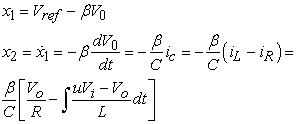(1)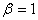In these equations V0, Vi, respectively stand for reference voltage, input voltage and the voltage sensed by voltage division by differentiating with respect to the equation related to x2.(2)

The parameter U presents the switching state, as U = 1 means switch is on and in contrast, U = 0, shows that switch is off.

2.1.1. Determining the Sliding Surface

To control the output voltage of the buck converter by sliding mode method, the circuit switching state (U) is determined by controlling parameters x1 and x2, by defining the sliding surface as in the following: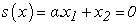(3)

In the equation above, the parameter α is called sliding coefficient which is calculated by applying Lyapunov stability law to the states on the sliding surface. By synthesizing the equations (1) and (3), the following equation for the sliding surface is obtained: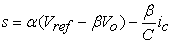(4)

But as we know, in such types of DC-DC converters, the capacitance is in the microfarad range and according to relation (4), its inverse term results in large coefficients for the circuit state variables (fed-back by the circuit) which practically leads to saturating the fed-back signals and causes transmitting error signals for the controller. To overcome this problem, a new redefinition of the sliding surface is proposed :(5)

Like the equation (4), but with a new definition of the sliding surface, we have:(6)

In the next section, with an appropriate definition for the parameter α, the sliding surface coefficients are independent from the C value and we will not have any more the problem of fed-back signal saturation.

2.1.2. Exerting (Implementing) the Sliding Condition

Equation (3) tracks the state variables remaining on the sliding surface toward the origin. To make sure of remaining the state variables on the sliding surface, the following condition, i.e. sliding condition must be obeyed: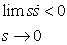(7)

This condition is derived from the Lyapanov law to examine the asymptotic stability of the systems controlled by the sliding mode . By differentiating with respect to time of the equation (3), and to obey the sliding condition in a small area in the vicinity of zero, we can write: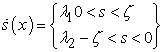(8)

Where ξ is a small positive number in the vicinity of zero and the parameters λ1 and λ2 are calculated by replacing the equation (3) with equations (2) and (4) :(9)

As a result, the best value of α to have the most operating point equals to:(10)

On the other hand, since the buck converter works like an isolated buck converter and furthermore, by drawing lines with the same slope for two open and closed states of the switch, the best value of α is calculated by equation (8).

2.1.3. Defining the Control Law

To control by sliding mode method in the second order systems where the switching state is determined by the controller, the phase trajectory behaviors around the sliding line can be observed to define the control law. Therefore, by use of Figure 2, the control law (switching state determination) is defined as follows: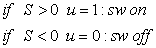(11)

But generally, if a converter is controlled by the sliding surface mode, the switching frequency corresponds to (is in compliance with) the movement oscillations frequency of the phase trajectory on the sliding line .

If these oscillations are uncontrollable, the controlled converter works with a high switching frequency which results in excessive switching losses, inductor and transformer core losses. According to the arguments on the non-linear controller, these oscillations are called chattering phenomenon and a presentation of hysteresis band with a definite width is required to overcome it . On the other hand, by producing (introducing) this bandwidth, the switching frequency can be controlled at the desired value. To create (introduce) the hysteresis band, the control law is changed as follows: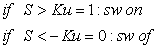(12)

In this control law, whenever the parameter S exceeds the bandwidth K, the switch is turned on and whenever it less than – K, it is turned off and between these two switching values, it stays in the previous state. In relation (5), K, is a small and positive value and to control the converter switching frequency, the relation between K and the switching frequency fs should be specified [9, 10]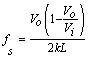(13)

2.1.6. Simulation and Data Analysis

The following simulation results are obtained by solving the state equations by the psim software and according to the data of the Table 1 in two states of desired k and calculated K.

#### Table 1. Specifications Of Buck ConverterDownload asPowerPoint Slide

Veiw figureView current table in a new window

According to relation (11), K value equals to 0.44 and therefore, the simulation is implemented for two states of bandwidth presence (k = 0.44) and lack of presence (k = 0).

Regarding the Figure 5-b, it can be claimed that by introducing the bandwidth to control the switching frequency, the reduction in switching frequency and the increase in steady state error in output voltage response can be seen. To reduce this error, it is important to limit k value to small values. On the other hand, k value should not be very small, since the very small values of the bandwidth results in excessive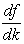, i.e. increase in frequency sensitivity to k changes. In other words, by an appropriate choice of k between increase of steady state error and decrease of switching losses, we choose one. According to , the desired k value is in the range of 0.1 < k < 0.2 and by choosing k = 0.136 and considering the switching frequency value, the L value is calculated. In fact, in equation (11), the practical L value is applied L (act) = 110.23 µH , which is naturally more than theoretical value and this causes the currency to stay in the continuous conduction mode (CCM).

Figure 5. Output voltage response for two values of k = 0.44 and k = 0 for (a) Transient response and (b) steady state response example

### 3. Examining the Transient Response with Change in Nominal Load Value

The output response to load increase at the moment of t = 0.25s

The output response to load increase at the moment of t= 0.25s.

As can be seen, by change in nominal (rating) load value, the dynamic response with optimal K in the hysteresis band is ameliorated.

### 4. Conclusion

In practical design and control of a buck converter by sliding mode method with definition of hysteresis band (k), it is required to choose one between the two steady state error and switching loss parameter. On the other hand, by choosing the optimal k and L (act) value, a relative balance between two parameters can be achieved. Moreover, by use of sliding mode control with nominal load change in the hysteresis band, a desired dynamic response id obtained.

### References

  Siew-Chong Tan,, Y. M. Lai, , Chi K. Tse, “A Fixed-Frequency Pulsewidth Modulation Based Quasi-Sliding-Mode Controller for Buck Converters” IEEE Trans. Power Electron, vol. 20, no. 6, pp. 1379-1392, Nov 2005.In article CrossRef  S.C. Tan, Y.M. Lai, M.K.H, Cheung, and C.K. Tse, “On the practical design of a sliding mode voltage controlled buck converter” IEEE Trans. Power Electron, vol. 20, no. 2, pp. 425-437, Mar 2005.In article CrossRef  G. Spiazzi and P. Mattavelli, “Sliding-mode control of switched-mode power supplies in The Power Electronics” Handbook. Boca Raton, FL: CRC Press LLC, 2002, ch. 8.In article  Hao-Ran Wang; Guo-Rong Zhu; Dong-Hua Zhang; Wei Chen; Yu Chen “On The Practical Design of a Single-Stage Single-Switch Isolated PFC Regulator Based on Sliding Mode Control” IEEE conf. Power Electron, vol. 1, pp. 719-724, 2012.In article  S.B. Guo, X.F. Lin-Shi, B. Allard, Y.X. Gao, and Y. Ruan, “Digital Sliding mode controller for high-frequency DC/DC SMPS,” IEEE Trans. Power Electron., vol. 25, no. 5, pp. 1120-1123, May 2010.In article CrossRef  R. Venkataramanan, A. Sabanoivc, and S. Cuk, “Sliding modecontrol of dc-to-dc converters,” in Proc. IEEE Conf. Industrial Electronics, Control Instrumentations (IECON), 1985, pp. 251-258.In article  V. M. Nguyen and C. Q. Lee, “Indirect implementations of slidingmodecontrol law in buck-type converters,” in Proc. IEEE Applied Power Electronics Conf. Expo (APEC), vol. 1, Mar. 1996, pp. 111-115.In article  P. Mattavelli, L. Rossetto, G. Spiazzi, and P. Tenti, “General-purposesliding-mode controller for dc / dc converter applications,” in Proc. IEEE Power Electronics Specialists Conf. (PESC), Jun. 1993, pp. 609-615.In article  Siew-Chong Tan; Lai, Y.M.; Tse, C.K.; An Adaptive Sliding Mode Controller for buck converterin Continuous Condition Mode IEEE Conf. Power Electron, vol. 3, pp. 1935-1400, 2004.In article  Yu Chen and Yong Kang, “The Variable-Bandwidth Hysteresis-Modulation Sliding-Mode Control for the PWM-PFM Converters” IEEE Trans. Power Electron., vol. 26, no. 10, pp. 2727-2734,Oct 2011.In article CrossRef